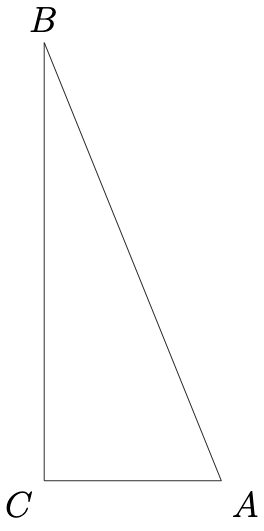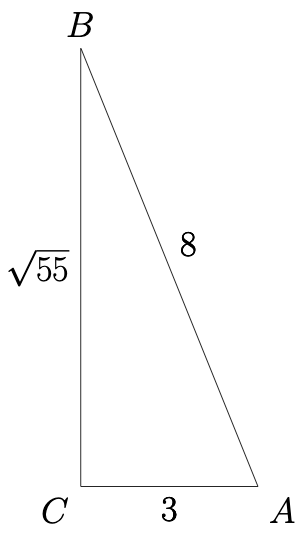# Finding an Angle’s Sine Given the Sine of Its Complement

This post about finding an angle’s sine given the sine of its complement is part of a series of posts to help you prepare for the Advanced Algebra and Functions part of the Accuplacer test.

## Question

Triangle ABC is a right triangle. Sin B = 3/8. What is the value of sin A?

## Solution

“Sin” is an abbreviation for sine. The sine of angle A in a right triangle means the ratio of the length of the side opposite angle A to the length of the hypotenuse (longest side).

You are asked to find an angle’s sine given the sine of its complement. A diagram should help. We can make some guesses about how triangle ABC looks. It’s conventional to name the right angle of a right triangle C, so right triangle ABC may look like this:Right triangle ABCRight triangle ABC.

That the sine of angle B is 3/8 means the length of the side opposite angle divided by the length of the hypotenuse is 3/8.

Those lengths may be 3 and 8 (or 30 and 80 or 0.3 and 0.8 or any combination in the proportion of 3/8).

The sine of angle is equal to the length of the side opposite angle A divided by the length of the hypotenuse. What is the length of the side opposite angle A?

### Sidebar: Pythagorean Theorem.

The Pythagorean Theorem states that the square of the length of the hypotenuse of a right triangle equals the sum of the squares of the lengths of the legs.Right triangle ABC

In other words, using the labels in the triangle at left, where each side is named for the angle opposite it, .

### Back to the Question

We need to know a. Solve the Pythagorean equation above for a:

And we know that and . Then

Now the triangle looks like this:Right triangle ABC.

The sine of A is the length of side opposite A divided by the length of the hypotenuse: